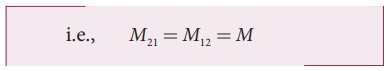Home | | Physics 12th Std | Mutual induction

# Mutual induction

When an electric current passing through a coil changes with time, an emf is induced in the neighbouring coil. This phenomenon is known as mutual induction and the emf is called mutually induced emf.

Mutual induction

When an electric current passing through a coil changes with time, an emf is induced in the neighbouring coil. This phenomenon is known as mutual induction and the emf is called mutually induced emf.Consider two coils which are placed close to each other. If an electric current i1 is sent through coil 1, the magnetic field produced by it is also linked with coil 2 as shown in Figure 4.22(a).

Let ╬”21 be the magnetic flux linked with each turn of the coil 2 of N2 turns due to coil 1, then the total flux linked with coil 2 ( N2 ╬” 21 ) is proportional to the current i1 in the coil 1.The constant of proportionality M21 is the mutual inductance of the coil 2 with respect to coil 1. It is also called as coefficient of mutual induction. If i1 =1A , then M 21 = N2 ╬” 21 . Therefore, the mutual inductance M21 is defined as the flux linkage of the coil 2 when 1A current flows through coil 1.

When the current i1 changes with time, an emf ╬Ą2 is induced in coil 2. From FaradayŌĆÖs law of electromagnetic induction, this mutually induced emf ╬Ą2 is given byThe negative sign in the above equation shows that the mutually induced emf always opposes the change in current i1 with respect to time. If di1/dt = 1 A s-1, then M21 = ŌłÆ ╬Ą .

Mutual inductance M21 is also defined as the opposing emf induced in the coil 2 when the rate of change of current through the coil 1 is 1 As-1.

Similarly, if an electric current i2 through coil 2 changes with time, then emf ╬Ą1 is induced in coil 1. Therefore,where M12 is the mutual inductance of the coil 1 with respect to coil 2. It can be shown that for a given pair of coils, the mutual inductance is same.In general, the mutual induction between two coils depends on size, shape, the number of turns of the coils, their relative orientation and permeability of the medium.

## Unit of mutual-inductance

The unit of mutual inductance is also henry (H).

If i1 =1A and N 2 ╬”21 =1 Wb turns, then M 21 =1H .

Therefore, the mutual inductance between two coils is said to be one henry if a current of 1A in coil 1 produces unit flux linkage in coil 2.

If di1/dt  = 1 AsŌłÆ1  and ╬Ą2 = ŌłÆ1 V , then M21 = 1H.

Therefore, the mutual inductance between two coils is one henry if a current changing at the rate of 1 As-1 in coil 1 induces an opposing emf of 1V in coil 2.

Tags : Definition, Explanation, Formula, Unit | Electromagnetic Induction , 12th Physics : Electromagnetic Induction and Alternating Current
Study Material, Lecturing Notes, Assignment, Reference, Wiki description explanation, brief detail
12th Physics : Electromagnetic Induction and Alternating Current : Mutual induction | Definition, Explanation, Formula, Unit | Electromagnetic Induction